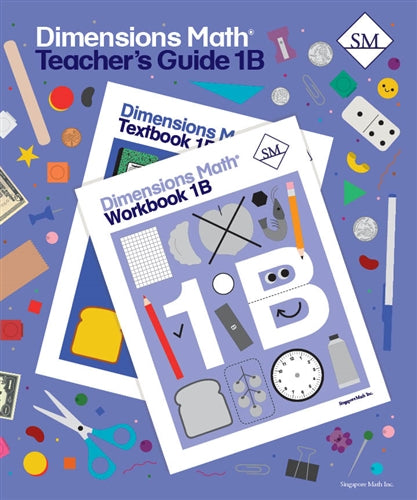# Dimensions Math Teacher's Guide 1B

\$ 25.00

Dimensions Math® Teacher's Guide 1B

Chapter 10: Length

Teaching Notes
Chapter Opener
Lesson 1: Comparing Lengths Directly
Lesson 2: Comparing Lengths Indirectly
Lesson 3: Comparing Lengths with Units
Lesson 4: Practice
Workbook Solutions

Chapter 11: Comparing

Teaching Notes
Chapter Opener
Lesson 1: Subtraction as Comparison
Lesson 2: Making Comparison Subtraction Stories
Lesson 3: Picture Graphs
Lesson 4: Practice
Workbook Solutions

Chapter 12: Numbers to 40

Teaching Notes
Chapter Opener
Lesson 1: Numbers to 40
Lesson 2: Tens and Ones
Lesson 3: Counting by Tens and Ones
Lesson 4: Comparing
Lesson 5: Practice
Workbook Solutions

Chapter 13: Addition and Subtraction Within 40

Teaching Notes
Chapter Opener
Lesson 2: Subtract Ones
Lesson 3: Make the Next Ten
Lesson 5: Subtract from Tens
Lesson 6: Use Subtraction Facts
Lesson 8: Practice
Workbook Solutions

Chapter 14: Grouping and Sharing

Teaching Notes
Chapter Opener
Lesson 2: Sharing
Lesson 3: Grouping
Lesson 4: Practice
Workbook Solutions

Chapter 15: FractionsTeaching Notes
Chapter Opener
Lesson 1: Halves
Lesson 2: Fourths
Lesson 3: Practice
Review 3
Workbook Solutions

Chapter 16: Numbers to 100

Teaching Notes
Chapter Opener
Lesson 1: Numbers to 100
Lesson 2: Tens and Ones
Lesson 3: Count by Ones or Tens
Lesson 4: Compare Numbers to 100
Lesson 5: Practice
Workbook Solutions

Chapter 17: Addition and Subtraction Within 100

Teaching Notes
Chapter Opener
Lesson 1: Add Ones — Part 1
Lesson 3: Add Ones — Part 2
Lesson 4: Add Tens and Ones — Part 1
Lesson 5: Add Tens and Ones — Part 2
Lesson 6: Subtract Ones — Part 1
Lesson 7: Subtract from Tens
Lesson 8: Subtract Ones — Part 2
Lesson 9: Subtract Tens
Lesson 10: Subtract Tens and Ones — Part 1
Lesson 11: Subtract Tens and Ones — Part 2
Lesson 12: Practice
Workbook Solutions

Chapter 18: Time

Teaching Notes
Chapter Opener
Lesson 1: Telling Time to the Hour
Lesson 2: Telling Time to the Half Hour
Lesson 3: Telling Time to the 5 Minutes
Lesson 4: Practice
Workbook Solutions

Chapter 19: Money

Teaching Notes
Chapter Opener
Lesson 1: Coins
Lesson 2: Counting Money
Lesson 3: Bills
Lesson 4: Shopping
Lesson 5: Practice
Review 4
Workbook Solutions

Blackline Masters for 1B

Dimensions Math® PreK-5 series features the progression, rigor, and pacing that define Singapore math. Throughout the series, five characters offer students suggestions on how to think about problems. They remind students of strategies they’ve learned and point out important information that encourages them to come up with their own solutions.

Textbook lessons begin with a task that allows students to apply their previous knowledge and learn through discussion. Once students have mastered a concept with the use of concrete and pictorial aids, they are ready to take on more abstract mathematical problem sets. They reach fluency by collecting various strategies along the way and applying them to new problems. Word problems give students a sense of math in real-world contexts.

Workbooks offer independent practice that follows a careful progression of exercise variation. Each textbook lesson includes a corresponding workbook exercise that starts with pictorial representation and progresses to more challenging abstract problems. Workbooks for PreK-2 are perforated.

Teacher’s Guides include lesson plans, mathematical background, games, helpful suggestions, and comprehensive resources for daily lessons. Lessons are laid out clearly and activities are designed for the whole class, small groups, and extension.

Textbooks and Workbooks do not include answer keys. Answers are in Teacher's Guides.
ISBN  9781947226333